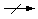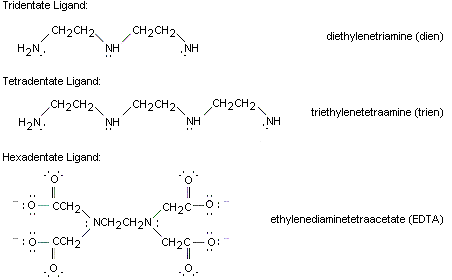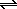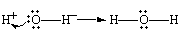Coordination Complexes and Ligands

Coordination Complexes and Ligands

Coordination Complexes

Coordination compounds, such as the FeCl4- ion and CrCl3 6 NH3, are called such because they contain ions or molecules linked, or coordinated, to a transition metal. They are also known as complex ions or coordination complexes because they are Lewis acid-base complexes. The ions or molecules that bind to transition-metal ions to form these complexes are called ligands (from Latin, "to tie or bind"). The number of ligands bound to the transition metal ion is called the coordination number.

Although coordination complexes are particularly important in the chemistry of the transition metals, some main group elements also form complexes. Aluminum, tin, and lead, for example, form complexes such as the AlF63-, SnCl42- and PbI42- ions.Werner's Theory of Coordination Complexes

Alfred Werner developed a model of coordination complexs which explains the following observations.

• At least three different cobalt(III) complexes can be isolated when CoCl2 is dissolved in aqueous ammonia and then oxidized by air to the +3 oxidation state. A fourth complex can be made by slightly different techniques. These complexes have different colors and different empirical formulas.
 CoCl3 6 NH3 orange-yellow CoCl3 5 NH3 H2O red CoCl3 5 NH3 purple CoCl3 4 NH3 green
• The reactivity of the ammonia in these complexes has been drastically reduced. By itself, ammonia reacts rapidly with hydrochloric acid to form ammonium chloride.

NH3(aq) + HCl(aq)NH4+(aq) + Cl-(aq)

These complexes don't react with hydrochloric acid, even at 100oC.

 CoCl3 6 NH3(aq) + HCl(aq)• Solutions of the Cl- ion react with Ag+ ion to form a white precipitate of AgCl.

Ag+(aq) + Cl-(aq)AgCl(s)

When excess Ag+ ion is added to solutions of the CoCl3 6 NH3 and CoCl3 5 NH3 H2O complexes, three moles of AgCl are formed for each mole of complex in solution, as might be expected. However, only two of the Cl- ions in the CoCl3 5 NH3 complex and only one of the Cl- ions in CoCl3 4 NH3 can be precipitated with Ag+ ions.

• Measurements of the conductivity of aqueous solutions of these complexes suggest that the CoCl3 6 NH3 and CoCl3 5 NH3 H2O complexes dissociate in water to give a total of four ions. CoCl3 5 NH3 dissociates to give three ions, and CoCl3 4 NH3 dissociates to give only two ions.

Werner explained these observations by suggesting that transition-metal ions such as the Co3+ ion have a primary valence and a secondary valence. The primary valence is the number of negative ions needed to satisfy the charge on the metal ion. In each of the cobalt(III) complexes previously described, three Cl- ions are needed to satisfy the primary valence of the Co3+ ion.

The secondary valence is the number of ions of molecules that are coordinated to the metal ion. Werner assumed that the secondary valence of the transition metal in these cobalt(III) complexes is six. The formulas of these compounds can therefore be written as follows.

 [Co(NH3)63+][Cl-]3 orange-yellow [Co(NH3)5(H2O)3+][Cl-]3 red [Co(NH3)5Cl2+][Cl-]2 purple [Co(NH3)4Cl2+][Cl-] green

The cobalt ion is coordinated to a total of six ligands in each complex, which satisfies the secondary valence of this ion. Each complex also has a total of three chloride ions that satisfy the primary valence. Some of the Cl- ions are free to dissociate when the complex dissolves in water. Others are bound to the Co3+ ion and neither dissociate nor react with Ag+.

The [Co(NH3)6]Cl3 complex dissociates in water to give a total of four ions, and all three Cl- ions are free to react with Ag+ ion.

 H2O [Co(NH3)6]Cl3(s)Co(NH3)63+(aq) + 3 Cl-(aq)

One of the chloride ions is bound to the cobalt in the [Co(NH3)5Cl]Cl2 complex. Only three ions are formed when this compound dissolves in water, and only two Cl- ions are free to precipitate with Ag+ ions.

 H2O [Co(NH3)5Cl][Cl]2(s)Co(NH3)5Cl2+(aq) + 2 Cl-(aq)

Once again, the three Cl- ions are free to dissociate when [Co(NH3)5(H2O)]Cl3 dissolves in water, and they precipitate when Ag+ ions are added to the solution.

 H2O [Co(NH3)5(H2O)]Cl3(s)Co(NH3)5(H2O)3+(aq) + 3 Cl-(aq)

Two of the chloride ions are bound to the cobalt in [Co(NH3)4Cl2]Cl. Only two ions are formed when this compound dissolves in water, and only one Cl- ion is free to precipitate with Ag+ ions.

 H2O [Co(NH3)4Cl2][Cl](s)Co(NH3)4Cl2+(aq) + Cl-(aq)

Werner assumed that transition-metal complexes had definite shapes. According to his theory, the ligands in six-coordinate cobalt(III) complexes are oriented toward the corners of an octahedron, as shown in the figure below.Typical Ligands

Any ion or molecule with a pair of nonbonding electrons can be a ligand. Many ligands are described as monodentate (literally, "one-toothed") because they "bite" the metal in only one place. Typical monodentate ligands are given in the figure below.Other ligands can attach to the metal more than once. Ethylenediamine (en) is a typical bidentate ligand.Each end of this molecule contains a pair of nonbonding electrons that can form a covalent bond to a metal ion. Ethylenediamine is also an example of a chelating ligand. The term chelate comes from a Greek stem meaning "claw." It is used to describe ligands that can grab the metal in two or more places, the way a claw would.

Linking ethylene- diamine fragments gives tridentate ligands and tetradentate ligands, such as diethylenetriamine (dien) and triethylenetetramine (trien). Adding four -CH2CO2- groups to an ethylenediamine framework gives a hexadentate ligand, which can single-handedly satisfy the secondary valence of a transition-metal ion.Transition-metal complexes have been characterized with coordination numbers that range from 1 to 12, but the most common coordination numbers are 2, 4, and 6. Examples of complexes with these coordination numbers are given in the table below.

Examples of Common Coordination Numbers

 Metal Ion Ligand Complex Coordination Number Ag+ + 2 NH3Ag(NH3)2+ 2 Ag+ + 2 S2O32-AgCl2- 2 Ag+ + 2 Cl-Ag(S2O3)23- 2 Pb2+ + 2 OAc-Pb(OAc)2 2 Cu+ + 2 NH3Cu(NH3)2+ 2 Cu2+ + 4 NH3Cu(NH3)42+ 4 Zn2+ + 4 CN-Zn(CN)42- 4 Hg2+ + 4 I-HgI42- 4 Co2+ + 4 SCN-Co(SCN)42- 4 Fe2+ + 6 H2OFe(H2O)62+ 6 Fe3+ + 6 H2OFe(H2O)63+ 6 Fe2+ + 6 CN-Fe(CN)64- 6 Co3+ + 6 NH3Co(NH3)63+ 6 Ni2+ + 6 NH3Ni(NH3)62+ 6

Note that the charge on the complex is always the sum of the charges on the ions or molecules that form the complex.

Cu2+ + 4 NH3Cu(NH3)42+

Pb2+ + 2 OAc-Pb(OAc)2

Fe2+ + 6 CN-Fe(CN)64-

Note also that the coordination number of a complex often increases as the charge on the metal ion becomes larger.

Cu+ + 2 NH3Cu(NH3)2+

Cu2+ + 4 NH3Cu(NH3)42+

 Practice Problem 2:Calculate the charge on the transition-metal ion in the following complexes. (a) Na2Co(SCN)4 (b) Ni(NH3)6(NO3)2 (c) K2PtCl6 Click here to check your answer to Practice Problem 2Lewis Acid-Lewis base Approach to Bonding in Complexes

G. N. Lewis was the first to recognize that the reaction between a transition-metal ion and ligands to form a coordination complex was analogous to the reaction between the H+ and OH- ions to form water. The reaction between H+ and OH- ions involves the donation of a pair of electrons from the OH- ion to the H+ ion to form a covalent bond.The H+ ion can be described as an electron-pair acceptor. The OH- ion, on the other hand, is an electron-pair donor. Lewis argued that any ion or molecule that behaves like the H+ ion should be an acid. Conversely, any ion or molecule that behaves like the OH- ion should be a base. A Lewis acid is therefore any ion or molecule that can accept a pair of electrons. A Lewis base is an ion or molecule that can donate a pair of electrons.

When Co3+ ions react with ammonia, the Co3+ ion accepts pairs of nonbonding electrons from six NH3 ligands to form covalent cobalt-nitrogen bonds as shown in the figure below.The metal ion is therefore a Lewis acid, and the ligands coordinated to this metal ion are Lewis bases.The Co3+ ion is an electron-pair acceptor, or Lewis acid, because it has empty valence-shell orbitals that can be used to hold pairs of electrons. To emphasize these empty valence orbitals we can write the configuration of the Co3+ ion as follows.

Co3+: [Ar] 3d6 4s0 4p0

There is room in the valence shell of this ion for 12 more electrons. (Four electrons can be added to the 3d subshell, two to the 4s orbital, and six to the 4p subshell.) The NH3 molecule is an electron-pair donor, or Lewis base, because it has a pair of nonbonding electrons on the nitrogen atom.

According to this model, transition-metal ions form coordination complexes because they have empty valence-shell orbitals that can accept pairs of electrons from a Lewis base. Ligands must therefore be Lewis bases: They must contain at least one pair of nonbonding electrons that can be donated to a metal ion.